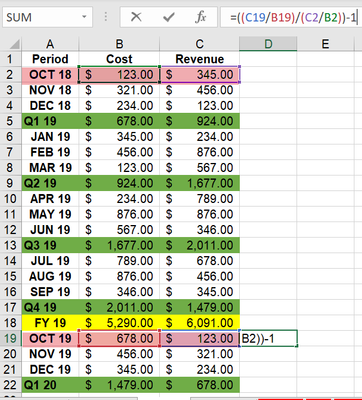cancel
Showing results for
Did you mean:Post Prodigy

## how to divide this month over last year same month data

Hi,

when I calculate Oct 19 %, I want it to be ((C19/B19)/(C2/B2))-1.

how to set it as this month revenue divide by this month cost over last month revenue divide by last month cost?

besides, how to calculate Quarter data with the same logic?1 ACCEPTED SOLUTIONSuper User

Hi,

Hope this helps.Regards,
Ashish Mathur
http://www.ashishmathur.com
7 REPLIES 7Super User

@PBI_newuser , Convert this month to date

Date = "01 " & [Month Year]

Then you can use time intelligence with date table.

MTD Sales = CALCULATE(SUM(Sales[Sales Amount]),DATESMTD('Date'[Date]))
last MTD Sales = CALCULATE(SUM(Sales[Sales Amount]),DATESMTD(dateadd('Date'[Date],-1,MONTH)))
last month Sales = CALCULATE(SUM(Sales[Sales Amount]),previousmonth('Date'[Date]))
this month =MTD Sales = CALCULATE(SUM(Sales[Sales Amount]),DATESMTD(ENDOFMONTH('Date'[Date])))

QTD Sales = CALCULATE(SUM(Sales[Sales Amount]),DATESQTD(('Date'[Date])))
Last QTD Sales = CALCULATE(SUM(Sales[Sales Amount]),DATESQTD(dateadd('Date'[Date],-1,QUARTER)))

Last complete QTD Sales = CALCULATE(SUM(Sales[Sales Amount]),DATESQTD( ENDOFQUARTER(dateadd('Date'[Date],-1,QUARTER))))
Last to last QTD Sales = CALCULATE(SUM(Sales[Sales Amount]),DATESQTD(dateadd('Date'[Date],-2,QUARTER)))

YTD Sales = CALCULATE(SUM(Sales[Sales Amount]),DATESYTD('Date'[Date],"12/31"))
Last YTD Sales = CALCULATE(SUM(Sales[Sales Amount]),DATESYTD(dateadd('Date'[Date],-1,Year),"12/31"))

Power BI — Year on Year with or Without Time Intelligence
https://medium.com/@amitchandak.1978/power-bi-ytd-questions-time-intelligence-1-5-e3174b39f38a
Power BI — Qtr on Qtr with or Without Time Intelligence
https://medium.com/@amitchandak.1978/power-bi-qtd-questions-time-intelligence-2-5-d842063da839
Power BI — Month on Month with or Without Time Intelligence
https://medium.com/@amitchandak.1978/power-bi-mtd-questions-time-intelligence-3-5-64b0b4a4090ePost Prodigy

Hi @amitchandak ,

How to calculate last year same month sales?

For example: Sales in Oct 19/ Sales in Oct 18Super User

Hi,

You will have to create a Calendar Table and build a relationship with the Date column in your Data Table.  In the Calendar Table, write calculated columns to extract Year, Quarter, Month Name and Month number.  Sort the Month name by the month number.  To your visual, drag Year, Month name and Quarter from the Calendar Table.  Thereafter DIVIDE(), SAMEPERIODLASTYEAR() functions will help you.

Regards,
Ashish Mathur
http://www.ashishmathur.comPost Prodigy

Below is the sample data.

How to keep those % value from Oct 18 to Sep 19 (FY19) as no calculation in the column?

How to calculate the % value from Oct 19 onwards, by monthly, quarterly and yearly?Super User

Hi,

Hope this helps.Regards,
Ashish Mathur
http://www.ashishmathur.comPost Prodigy

Thanks @Ashish_Mathur !!
It works!Super User

You are welcome.

Regards,
Ashish Mathur
http://www.ashishmathur.com